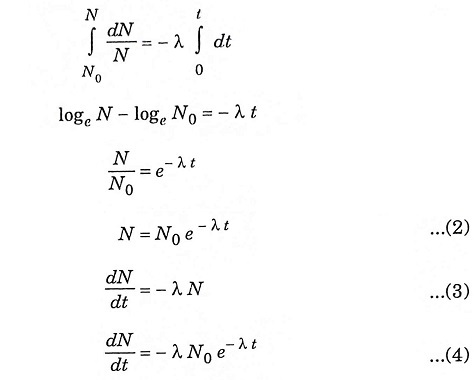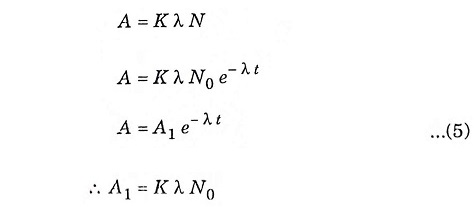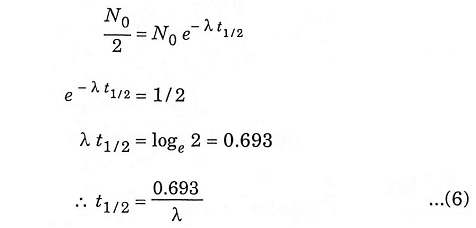## What is Radioactivity? – Definition and Types

The phenomenon of spontaneous emission of powerful radiation exhibited by heavy elements is called radioactivity. It is an irreversible self-disintegrating activity as the elements breaks itself up (fission reaction). The elements that exhibit this activity are called radioactive elements. Example of radioactive elements are uranium, radium, thorium, pulutonium, radon, ionium, actinium and mesothorium.

The radioactivity may be natural or artificial. Natural radioactivity is exhibited by elements as found in nature and occurs generally in heavier elements of the periodic table, whereas artificial radioactivity is a modern technique of transmutation of elements that are lighter than those elements that exhibits radioactivity naturally.

1. α (Alpha) particle
2. β (Beta) particle
3. γ (Gamma) rays (or) photons

### α particles

These are helium nuclei consisting of two protons and two neutrons emitted from both natural and artificial radioactivity. They are highly ionised but cannot penetrate the skin, so are dangerous only if emitted inside the body.

### β – particles

These are fast moving electron emitted by radioactive elements. They are more penetrating than α – particles, but can be easily shielded by a few millimeter of wood or aluminium. They can penetrate a little way into human flesh but are generally less dangerous to people than γ – rays. Its exposure results in effects like sunburn, but it is slower to heal.

### γ ray

These are high-energy beam much the same as X-rays. They are excited in many radioactive decay and may be very penetrating, therefore requires much substantial shielding. γ – rays are the main hazard to people dealing with sealed radioactive materials used.

### Effects of nuclear radiation on matter

When nuclear radiation interacts with any materials, deposits energy in the materials and have various effects. The chemical properties get altered, the crystalline structure may be altered.

The emission of the particles in the form of alpha, beta and gamma radiation is not an instantaneous process. For different element the decay time is different, which follows a certain law. The process is independent of the physical and chemical properties of the given isotope at a particular temperature and pressure.

The law states that “small amount of disintegration of the isotope in a small period is directly proportional to the total number or radioactive nuclei and proportionality constant”.

The above law can be stated in form of equation aswhere

• N = Total number of nuclei present
• dN = Number of nuclei present at time dt
• dt = Small time interval
• λ = Proportionality constant

Integrating equation (1) with limits, we get### Activity

The intensity of emitted radiation is termed activity. This is directly proportional to the rate of disintegration of the element.

Let A = activity of time tFrom equation (3) and (4)when

• A1 = initial activity
• K= detection coefficient

### Half life

Half-life is the time required for half of the parent nuclei to decay.

Putting N = N0/2 and t = t1/2 in equation (2), we get### Average life

Average life denotes the average of total time for which the radioactive nuclei has disintegrated for several half life. It is greater then the half life.

Let T = Average life, then

Scroll to Top